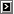# erikbTECH
Calculate the number of days between two dates in PHP this [2006-10-17]

Not exactly wizard code, but why not publish it anyway... I googled for an example like this myself but had trouble finding a good match. Maybe it was more a question of finding the right keywords to search for.

I use this code on the Swedish site for the World Usability Day.

```function daystogo() {
// my target date is November 14th 2006
\$then = mktime(0, 0, 0, 11, 14, 2006);
// calculate difference between now and then
\$now = time();
\$diff = \$then - \$now;
// second, minute, hour
\$days = round(0.5 + \$diff / 60 / 60 / 24);
// make sure we do not return negative zeros
if (\$days == -0) \$days = 0;

return \$days;
}
// somewhere later in the code...
\$days = daystogo();
// if (\$days > 0) ...
```